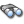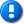home | career | drupal | java | mac | mysql | perl | scala | uml | unix

# Java example source code file (AbstractLeastSquaresOptimizerTestValidation.java)

This example Java source code file (AbstractLeastSquaresOptimizerTestValidation.java) is included in the alvinalexander.com "Java Source Code Warehouse" project. The intent of this project is to help you "Learn Java by Example" TM.

Learn more about this Java project at its project page.

## Java - Java tags/keywords

abstractleastsquaresoptimizer, arraylist, awt, deprecated, dummyoptimizer, geometry, monte_carlo_runs, pointvectorvaluepair, randomstraightlinepointgenerator, should, statisticalsummary, straightlineproblem, string, summarystatistics, test, util, xxx

## The AbstractLeastSquaresOptimizerTestValidation.java Java example source code

```/*
* Licensed to the Apache Software Foundation (ASF) under one or more
* contributor license agreements. See the NOTICE file distributed with this
* work for additional information regarding copyright ownership. The ASF
* licenses this file to You under the Apache License, Version 2.0 (the
* "License"); you may not use this file except in compliance with the License.
* You may obtain a copy of the License at
* http://www.apache.org/licenses/LICENSE-2.0 Unless required by applicable law
* or agreed to in writing, software distributed under the License is
* distributed on an "AS IS" BASIS, WITHOUT WARRANTIES OR CONDITIONS OF ANY
* KIND, either express or implied. See the License for the specific language
* governing permissions and limitations under the License.
*/
package org.apache.commons.math3.optimization.general;

import java.util.Arrays;
import java.util.List;
import java.util.ArrayList;
import java.awt.geom.Point2D;

import org.apache.commons.math3.optimization.PointVectorValuePair;
import org.apache.commons.math3.stat.descriptive.SummaryStatistics;
import org.apache.commons.math3.stat.descriptive.StatisticalSummary;
import org.apache.commons.math3.util.FastMath;
import org.junit.Test;
import org.junit.Assert;

/**
* This class demonstrates the main functionality of the
* {@link AbstractLeastSquaresOptimizer}, common to the
* optimizer implementations in package
* {@link org.apache.commons.math3.optimization.general}.
* <br/>
* Not enabled by default, as the class name does not end with "Test".
* <br/>
* Invoke by running
* <pre>```
*  mvn test -Dtest=AbstractLeastSquaresOptimizerTestValidation
* </code>``````
``` * or by running * <pre> * mvn test -Dtest=AbstractLeastSquaresOptimizerTestValidation -DargLine="-DmcRuns=1234 -server" * </code> */ @Deprecated public class AbstractLeastSquaresOptimizerTestValidation { private static final int MONTE_CARLO_RUNS = Integer.parseInt(System.getProperty("mcRuns", "100")); /** * Using a Monte-Carlo procedure, this test checks the error estimations * as provided by the square-root of the diagonal elements of the * covariance matrix. * <br/> * The test generates sets of observations, each sampled from * a Gaussian distribution. * <br/> * The optimization problem solved is defined in class * {@link StraightLineProblem}. * <br/> * The output (on stdout) will be a table summarizing the distribution * of parameters generated by the Monte-Carlo process and by the direct * estimation provided by the diagonal elements of the covariance matrix. */ @Test public void testParametersErrorMonteCarloObservations() { // Error on the observations. final double yError = 15; // True values of the parameters. final double slope = 123.456; final double offset = -98.765; // Samples generator. final RandomStraightLinePointGenerator lineGenerator = new RandomStraightLinePointGenerator(slope, offset, yError, -1e3, 1e4, 138577L); // Number of observations. final int numObs = 100; // XXX Should be a command-line option. // number of parameters. final int numParams = 2; // Parameters found for each of Monte-Carlo run. final SummaryStatistics[] paramsFoundByDirectSolution = new SummaryStatistics[numParams]; // Sigma estimations (square-root of the diagonal elements of the // covariance matrix), for each Monte-Carlo run. final SummaryStatistics[] sigmaEstimate = new SummaryStatistics[numParams]; // Initialize statistics accumulators. for (int i = 0; i < numParams; i++) { paramsFoundByDirectSolution[i] = new SummaryStatistics(); sigmaEstimate[i] = new SummaryStatistics(); } // Dummy optimizer (to compute the covariance matrix). final AbstractLeastSquaresOptimizer optim = new DummyOptimizer(); final double[] init = { slope, offset }; // Monte-Carlo (generates many sets of observations). final int mcRepeat = MONTE_CARLO_RUNS; int mcCount = 0; while (mcCount < mcRepeat) { // Observations. final Point2D.Double[] obs = lineGenerator.generate(numObs); final StraightLineProblem problem = new StraightLineProblem(yError); for (int i = 0; i < numObs; i++) { final Point2D.Double p = obs[i]; problem.addPoint(p.x, p.y); } // Direct solution (using simple regression). final double[] regress = problem.solve(); // Estimation of the standard deviation (diagonal elements of the // covariance matrix). final PointVectorValuePair optimum = optim.optimize(Integer.MAX_VALUE, problem, problem.target(), problem.weight(), init); final double[] sigma = optim.computeSigma(optimum.getPoint(), 1e-14); // Accumulate statistics. for (int i = 0; i < numParams; i++) { paramsFoundByDirectSolution[i].addValue(regress[i]); sigmaEstimate[i].addValue(sigma[i]); } // Next Monte-Carlo. ++mcCount; } // Print statistics. final String line = "--------------------------------------------------------------"; System.out.println(" True value Mean Std deviation"); for (int i = 0; i < numParams; i++) { System.out.println(line); System.out.println("Parameter #" + i); StatisticalSummary s = paramsFoundByDirectSolution[i].getSummary(); System.out.printf(" %+.6e %+.6e %+.6e\n", init[i], s.getMean(), s.getStandardDeviation()); s = sigmaEstimate[i].getSummary(); System.out.printf("sigma: %+.6e (%+.6e)\n", s.getMean(), s.getStandardDeviation()); } System.out.println(line); // Check the error estimation. for (int i = 0; i < numParams; i++) { Assert.assertEquals(paramsFoundByDirectSolution[i].getSummary().getStandardDeviation(), sigmaEstimate[i].getSummary().getMean(), 8e-2); } } /** * In this test, the set of observations is fixed. * Using a Monte-Carlo procedure, it generates sets of parameters, * and determine the parameter change that will result in the * normalized chi-square becoming larger by one than the value from * the best fit solution. * <br/> * The optimization problem solved is defined in class * {@link StraightLineProblem}. * <br/> * The output (on stdout) will be a list of lines containing: * <ul> * <li>slope of the straight line, * <li>intercept of the straight line, * <li>chi-square of the solution defined by the above two values. * </ul> * The output is separated into two blocks (with a blank line between * them); the first block will contain all parameter sets for which * {@code chi2 < chi2_b + 1} * and the second block, all sets for which * {@code chi2 >= chi2_b + 1} * where {@code chi2_b} is the lowest chi-square (corresponding to the * best solution). */ @Test public void testParametersErrorMonteCarloParameters() { // Error on the observations. final double yError = 15; // True values of the parameters. final double slope = 123.456; final double offset = -98.765; // Samples generator. final RandomStraightLinePointGenerator lineGenerator = new RandomStraightLinePointGenerator(slope, offset, yError, -1e3, 1e4, 13839013L); // Number of observations. final int numObs = 10; // number of parameters. // Create a single set of observations. final Point2D.Double[] obs = lineGenerator.generate(numObs); final StraightLineProblem problem = new StraightLineProblem(yError); for (int i = 0; i < numObs; i++) { final Point2D.Double p = obs[i]; problem.addPoint(p.x, p.y); } // Direct solution (using simple regression). final double[] regress = problem.solve(); // Dummy optimizer (to compute the chi-square). final AbstractLeastSquaresOptimizer optim = new DummyOptimizer(); // Get chi-square of the best parameters set for the given set of // observations. final double bestChi2N = getChi2N(optim, problem, regress); final double[] sigma = optim.computeSigma(regress, 1e-14); // Monte-Carlo (generates a grid of parameters). final int mcRepeat = MONTE_CARLO_RUNS; final int gridSize = (int) FastMath.sqrt(mcRepeat); // Parameters found for each of Monte-Carlo run. // Index 0 = slope // Index 1 = offset // Index 2 = normalized chi2 final List<double[]> paramsAndChi2 = new ArrayList(gridSize * gridSize); final double slopeRange = 10 * sigma; final double offsetRange = 10 * sigma; final double minSlope = slope - 0.5 * slopeRange; final double minOffset = offset - 0.5 * offsetRange; final double deltaSlope = slopeRange/ gridSize; final double deltaOffset = offsetRange / gridSize; for (int i = 0; i < gridSize; i++) { final double s = minSlope + i * deltaSlope; for (int j = 0; j < gridSize; j++) { final double o = minOffset + j * deltaOffset; final double chi2N = getChi2N(optim, problem, new double[] {s, o}); paramsAndChi2.add(new double[] {s, o, chi2N}); } } // Output (for use with "gnuplot"). // Some info. // For plotting separately sets of parameters that have a large chi2. final double chi2NPlusOne = bestChi2N + 1; int numLarger = 0; final String lineFmt = "%+.10e %+.10e %.8e\n"; // Point with smallest chi-square. System.out.printf(lineFmt, regress, regress, bestChi2N); System.out.println(); // Empty line. // Points within the confidence interval. for (double[] d : paramsAndChi2) { if (d <= chi2NPlusOne) { System.out.printf(lineFmt, d, d, d); } } System.out.println(); // Empty line. // Points outside the confidence interval. for (double[] d : paramsAndChi2) { if (d > chi2NPlusOne) { ++numLarger; System.out.printf(lineFmt, d, d, d); } } System.out.println(); // Empty line. System.out.println("# sigma=" + Arrays.toString(sigma)); System.out.println("# " + numLarger + " sets filtered out"); } /** * @return the normalized chi-square. */ private double getChi2N(AbstractLeastSquaresOptimizer optim, StraightLineProblem problem, double[] params) { final double[] t = problem.target(); final double[] w = problem.weight(); optim.optimize(Integer.MAX_VALUE, problem, t, w, params); return optim.getChiSquare() / (t.length - params.length); } } /** * A dummy optimizer. * Used for computing the covariance matrix. */ @Deprecated class DummyOptimizer extends AbstractLeastSquaresOptimizer { public DummyOptimizer() { super(null); } /** * This method does nothing and returns a dummy value. */ @Override public PointVectorValuePair doOptimize() { final double[] params = getStartPoint(); final double[] res = computeResiduals(computeObjectiveValue(params)); setCost(computeCost(res)); return new PointVectorValuePair(params, null); } } Other Java examples (source code examples) Here is a short list of links related to this Java AbstractLeastSquaresOptimizerTestValidation.java source code file:The search pageOther Java source code examples at this package levelClick here to learn more about this project ```

Copyright 1998-2021 Alvin Alexander, alvinalexander.com
All Rights Reserved.

A percentage of advertising revenue from
pages under the /java/jwarehouse URI on this website is
paid back to open source projects.